﻿ 有理Bézier曲线及其应用 Rational Bézier Curve and Its Application

Vol.06 No.08(2017), Article ID:22765,7 pages
10.12677/AAM.2017.68112

Rational Bézier Curve and Its Application

Fang Wang, Genzhu Bai

1Zhejiang Changzheng Vocational & Technical College, Hangzhou Zhejiang

2Inner Mongolia University for the Nationalities, Tongliao Inner Mongolia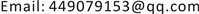Received: Nov. 3rd, 2017; accepted: Nov. 16th, 2017; published: Nov. 23rd, 2017ABSTRACT

Our study introduces rational Bézier curves to blend the axes of two tubes with non-coplanar axes. Furthermore, our method constructs blended tubes with the same radius but non-coplanar axes. By choosing appropriate weight factors for the corresponding control vertices, we can control the distances between the axes and the control polygons. Our method could be favorable in application.

Keywords:Rational Bézier Curves, Blending, Axes, Tubes

1浙江长征职业技术学院，浙江 杭州

2内蒙古民族大学，内蒙古 通辽1. 引言

$CY{L}_{1}$$CY{L}_{2}$ 为以 ${L}_{1}$${L}_{2}$ 为轴线的给定二管道的参数表示， ${P}_{1},{P}_{2}$ 为给定的平面的参数表示，它们确定的曲面分别表示为 $s\left(CY{L}_{i}\right)\left(i=1,2\right)$ ，其中 $s\left({P}_{i},CY{L}_{i}\right)\left(i=1,2\right)$ 分别为柱面和平面的交线。

$s\left({P}_{i}\right)\left(i=1,2\right)$ 为与 $s\left(CY{L}_{i}\right)\left(i=1,2\right)$ 的轴线垂直的平面，则

$\begin{array}{l}{P}_{1}={A}_{1}\left(x-{X}_{1}\right)+{B}_{1}\left(y-{Y}_{1}\right)+{C}_{1}\left(z-{Z}_{1}\right),\\ {P}_{2}={A}_{2}\left(x-{X}_{2}\right)+{B}_{2}\left(y-{Y}_{2}\right)+{C}_{2}\left(z-{Z}_{2}\right).\end{array}$

2. 有理Bézier曲线及其性质

$r\left(t\right)=\underset{i=0}{\overset{n}{\sum }}{B}_{n,i}\left(t\right){V}_{i},$

$r\left(t\right)=\frac{\underset{i=0}{\overset{n}{\sum }}{B}_{n,i}\left(t\right){w}_{i}{V}_{i}}{\underset{i=0}{\overset{n}{\sum }}{B}_{n,i}\left(t\right){w}_{i}}$

1) 若权因子有正、有负，则出现数值不稳定现象；

2) 若权因子同号并取互为相反的两组数，所得有理Bézier曲线重叠，以下假设 ${w}_{i}>0,i=0,1,\cdots ,n$

3) 若所有权因子取相同的数 ${w}_{1}={w}_{2}=\cdots ={w}_{n}=k>0$ ，则有理Bézier曲线退化为Bézier曲线；

4) 若 $0<{w}_{i}<1,i=0,1,\cdots ,n$ ，则所得有理Bézier曲线远离控制多边形；

5) 若 ${w}_{i}>1,i=0,1,\cdots ,n$ ，则所得Bézier曲线靠近控制多边形，见图1图2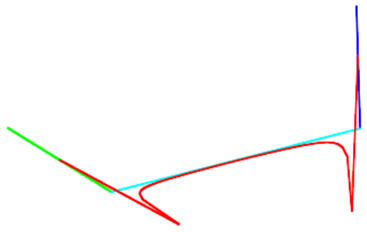Figure 1. (Case of ${w}_{0}=1,{w}_{1}={w}_{2}=-30,{w}_{3}=1$ and ${w}_{0}=1,{w}_{1}=-2,{w}_{2}=20,{w}_{3}=-3$ )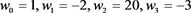Figure 2. (Case of ${w}_{0}={w}_{1}={w}_{2}={w}_{3}=\frac{1}{2}$ and ${w}_{0}=2,{w}_{1}=20,{w}_{2}=20,{w}_{3}=2$ )

3. 构造基于轴线光滑拼接的管道拼接曲面

${L}_{1}:\left\{\begin{array}{l}x={X}_{1}+{A}_{1}s,\\ y={Y}_{1}+{B}_{1}s,\\ z={Z}_{1}+{C}_{1}s.\end{array}$${L}_{2}:\left\{\begin{array}{l}x={X}_{2}+{A}_{2}s,\\ y={Y}_{2}+{B}_{2}s,\\ z={Z}_{2}+{C}_{2}s.\end{array}$ 为两个给定管道的轴线的参数方程，这里的直线 ${l}_{1}$ 是过点

${O}_{1}\left({X}_{1},{Y}_{1},{Z}_{1}\right)$ 且方向矢量为 ${d}_{1}\left({A}_{1},{B}_{1},{C}_{1}\right)$${l}_{2}$ 是过点 ${O}_{2}\left({X}_{2},{Y}_{2},{Z}_{2}\right)$ 且方向矢量为 ${d}_{2}\left({A}_{2},{B}_{2},{C}_{2}\right)$ 。则空间两直线 ${l}_{1}$${l}_{2}$ 是异面直线的充要条件为

$|\begin{array}{ccc}{X}_{2}-{X}_{1}& {Y}_{2}-{Y}_{1}& {Z}_{2}-{Z}_{1}\\ {A}_{1}& {B}_{1}& {C}_{1}\\ {A}_{2}& {B}_{2}& {C}_{2}\end{array}|\ne 0.$

$CY{L}_{1}$$CY{L}_{2}$ 为给定的二管道的参数表示， ${P}_{1},{P}_{2}$ 为给定的平面的参数表示，它们确定的曲面分别表示为 $s\left(CY{L}_{i}\right)$$s\left({P}_{i}\right)\left(i=1,2\right)$$s\left(CY{L}_{i},{P}_{i}\right)\left(i=1,2\right)$ 为管道和平面的交线。

${L}_{1}:\left\{\begin{array}{l}x={x}_{1}+0s,\\ y={y}_{1}+{B}_{1}s,\\ z=0+0s.\end{array}$${L}_{2}:\left\{\begin{array}{l}x=0+0s,\\ y={y}_{2}+0s,\\ z={z}_{2}+{C}_{2}s.\end{array}$

$\left\{\begin{array}{l}x={X}_{1}+a\mathrm{cos}t+a\mathrm{sin}t,\\ y={Y}_{1}+{B}_{1}s+a\mathrm{cos}t+a\mathrm{sin}t,\\ z=a\mathrm{cos}t+a\mathrm{sin}t.\end{array}$$t\in \left[0,2\text{π}\right]$ 其中 $a$ 为管道的半径。在这种假设下，先构造光滑拼接轴线的曲线，进而构造光滑拼接两个轴线异面的管道的拼接曲面。

$\left\{\begin{array}{l}X=\frac{\left(-{u}^{3}+3{u}^{2}-3u+1\right){w}_{0}{x}_{0}+\left(3{u}^{3}-6{u}^{2}+3u\right){w}_{1}{x}_{1}+\left(-3{u}^{3}+3{u}^{2}\right){w}_{2}{x}_{2}+{u}^{3}{w}_{3}{x}_{3}}{\left(-{u}^{3}+3{u}^{2}-3u+1\right){w}_{0}+\left(3{u}^{3}-6{u}^{2}+3u\right){w}_{1}+\left(-3{u}^{3}+3{u}^{2}\right){w}_{2}+{u}^{3}{w}_{3}},\\ Y=\frac{\left(-{u}^{3}+3{u}^{2}-3u+1\right){w}_{0}{y}_{0}+\left(3{u}^{3}-6{u}^{2}+3u\right){w}_{1}{y}_{1}+\left(-3{u}^{3}+3{u}^{2}\right){w}_{2}{y}_{2}+{u}^{3}{w}_{3}{y}_{3}}{\left(-{u}^{3}+3{u}^{2}-3u+1\right){w}_{0}+\left(3{u}^{3}-6{u}^{2}+3u\right){w}_{1}+\left(-3{u}^{3}+3{u}^{2}\right){w}_{2}+{u}^{3}{w}_{3}},\\ Z=\frac{\left(-{u}^{3}+3{u}^{2}-3u+1\right){w}_{0}{z}_{0}+\left(3{u}^{3}-6{u}^{2}+3u\right){w}_{1}{z}_{1}+\left(-3{u}^{3}+3{u}^{2}\right){w}_{2}{z}_{2}+{u}^{3}{w}_{3}{z}_{3}}{\left(-{u}^{3}+3{u}^{2}-3u+1\right){w}_{0}+\left(3{u}^{3}-6{u}^{2}+3u\right){w}_{1}+\left(-3{u}^{3}+3{u}^{2}\right){w}_{2}+{u}^{3}{w}_{3}}.\end{array}$

$p\left(u,t\right)=\left\{\begin{array}{l}X+a{N}_{1}\left(u\right)\mathrm{cos}t+a{B}_{1}\left(u\right)\mathrm{sin}t,\\ Y+a{N}_{2}\left(u\right)\mathrm{cos}t+a{B}_{2}\left(u\right)\mathrm{sin}t,\\ Z+a{N}_{3}\left(u\right)\mathrm{cos}t+a{B}_{3}\left(u\right)\mathrm{sin}t.\end{array}$

4. 拼接实例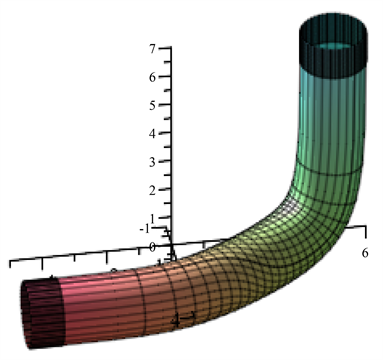Figure 3. Blending of two tubes with non-coplanar axes when the dihedral angle pi/2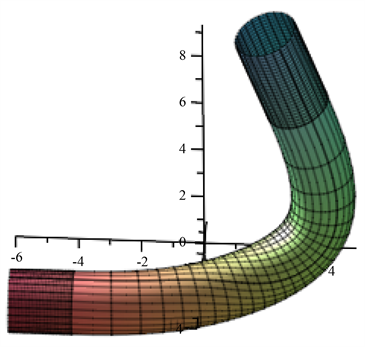Figure 4. Blending of two tubes with non-coplanar axes when the dihedral angle is smaller than pi/2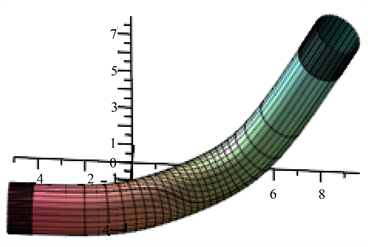Figure 5. Blending of two tubes with non-coplanar axes when the dihedral angle is larger than pi/2

5. 结束语

Rational Bézier Curve and Its Application[J]. 应用数学进展, 2017, 06(08): 935-941. http://dx.doi.org/10.12677/AAM.2017.68112

1. 1. Warren, J. (1989) Blending Algebraic Surfaces. ACM Transactions on Graphics, 8, 263-278.
https://doi.org/10.1145/77269.77270

2. 2. 吴文俊, 王定康. CAGD中的代数曲面拟合问题[J]. 数学的实践与认识, 1994(3): 26-31.

3. 3. Wallner, J. and Pottmann, H. (1997) Rational Blending Surfaces between Quadrics. CAGD, 14, 407-419.

4. 4. Wu, T.R. and Zhou. Y.S. (2000) On Blending of Several Quadratic Algebraic Surfaces. CAGD, 17, 759-766.

5. 5. Cheng, J.S. (2003) Blending Quadric Surfaces via a Base Curve Method. In: Proceedings of ASCM03, World Scientific, Beijing, 17-19 April 2003, 77-86.
https://doi.org/10.1142/9789812704436_0007

6. 6. 雷娜, 崔丽, 伍铁如. 两个轴异面的管道拼接[J]. 吉林大学学报(理学版), 2002, 40(2): 138-140.

7. 7. 白根柱. 两个轴异面圆柱面的光滑拼接问题[D]: [硕士学位论文]. 长春: 吉林大学, 2004.

8. 8. 白根柱. 隐式代数曲面拼接[J]. 数学的实践与认识, 2006, 36(4): 274-277.

9. 9. Hartmann, E. (2001) Gn-Continuous Connections between Normal Ringed Surfaces. Computer Aided Geometric Design, 18, 751-770.

10. 10. Bai, G.Z., Wang, H. and Yin, Z.J. (2014) Employing Generalized Bézier Tube to Smoothly Blending Tubes Whose Axes Are Non-Coplanar. Applied Mechanics and Mate-rials, 513-517, 2301-2306.

11. 11. Wang, H. and Bai, G.Z. (2013) Employing Generalized Cylindrical Helicoid Tube to Smoothly Blending Tubes Whose Axes are Non-Coplanar. Applied Mechanics and Materials, 380-384, 1750-1754.

12. 12. Bai, G.Z. and Wu, Z. (2013) Multi-Degree Reduction Approximation of Bézier Curves by Subdivision Method. Intelligent Information Management Systems and Technologies, 9, 37-42.

13. 13. Bai, G.Z., Liu, S.Y., Wang, H., Wu, Z. and Yin, Z.J. (2014) A Novel Method for Smooth Blending Cylindrical Surfaces Whose Axes Are Non-Coplanar Based on Smooth Blending Axes. Applied Mechanics and Materials, 687-691, 1470-1473.

14. 14. Bai, G.Z., Wang, H., Wu, Z., Yin, Z.J. and Liu, S.Y. (2015) Smoothly Blending of Two Elliptic Cylinders Whose Axes are Non-Coplanar. Applied Mechanics and Materials, 713-715, 152-158.

15. 15. 刘斯语, 白根柱. 利用推广的Hartmann方法光滑拼接两个异面直线[J]. 内蒙古民族大学学报(自然科学版), 2015(2): 102-104.

16. 16. 邱梦鸾, 肖琳, 白根柱. 用单页双曲螺旋管实现两个轴异面管道的光滑拼接[J]. 重庆师范大学学报(自然科学版), 2016(2): 108-112.